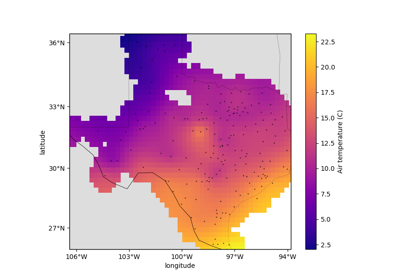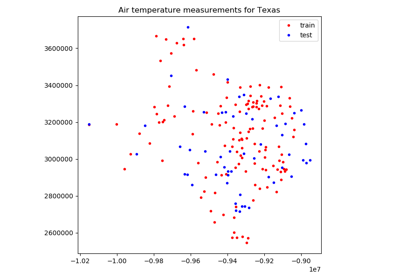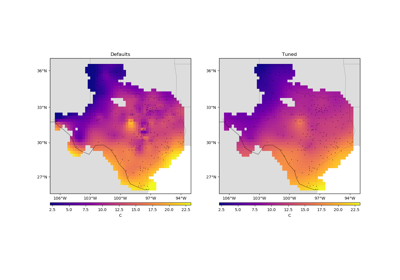# verde.cross_val_score¶

verde.cross_val_score(estimator, coordinates, data, weights=None, cv=None, client=None, delayed=False)[source]

Score an estimator/gridder using cross-validation.

Similar to sklearn.model_selection.cross_val_score but modified to accept spatial multi-component data with weights.

By default, will use sklearn.model_selection.KFold with n_splits=5 and random_state=0 to split the dataset. Any other cross-validation class can be passed in through the cv argument.

Can optionally run in parallel using dask. To do this, use delayed=True to dispatch computations with dask.delayed instead of running them. The returned scores will be “lazy” objects instead of the actual scores. To trigger the computation (which Dask will run in parallel) call the .compute() method of each score or dask.compute with the entire list of scores.

Warning

The client parameter is deprecated and will be removed in Verde v2.0.0. Use delayed instead.

Parameters
• estimator (verde gridder) – Any verde gridder class that has the fit and score methods.

• coordinates (tuple of arrays) – Arrays with the coordinates of each data point. Should be in the following order: (easting, northing, vertical, …).

• data (array or tuple of arrays) – the data values of each data point. If the data has more than one component, data must be a tuple of arrays (one for each component).

• weights (none or array or tuple of arrays) – if not none, then the weights assigned to each data point. If more than one data component is provided, you must provide a weights array for each data component (if not none).

• cv (None or cross-validation generator) – Any scikit-learn cross-validation generator. Defaults to sklearn.model_selection.KFold.

• client (None or dask.distributed.Client) – DEPRECATED: This option is deprecated and will be removed in Verde v2.0.0. If None, then computations are run serially. Otherwise, should be a dask Client object. It will be used to dispatch computations to the dask cluster.

• delayed (bool) – If True, will use dask.delayed to dispatch computations without actually executing them. The returned scores will be a list of delayed objects. Call .compute() on each score or dask.compute on the entire list to trigger the actual computations.

Returns

scores (array) – Array of scores for each split of the cross-validation generator. If delayed, will be a list of Dask delayed objects (see the delayed option). If client is not None, then the scores will be futures.

Examples

As an example, we can score verde.Trend on data that actually follows a linear trend.

>>> from verde import grid_coordinates, Trend
>>> coords = grid_coordinates((0, 10, -10, -5), spacing=0.1)
>>> data = 10 - coords + 0.5*coords
>>> model = Trend(degree=1)


In this case, the model should perfectly predict the data and R² scores should be equal to 1.

>>> scores = cross_val_score(model, coords, data)
>>> print(', '.join(['{:.2f}'.format(score) for score in scores]))
1.00, 1.00, 1.00, 1.00, 1.00


There are 5 scores because the default cross-validator is sklearn.model_selection.KFold with n_splits=5.

We can use different cross-validators by assigning them to the cv argument:

>>> from sklearn.model_selection import ShuffleSplit
>>> # Set the random state to get reproducible results
>>> cross_validator = ShuffleSplit(n_splits=3, random_state=0)
>>> scores = cross_val_score(model, coords, data, cv=cross_validator)
>>> print(', '.join(['{:.2f}'.format(score) for score in scores]))
1.00, 1.00, 1.00


If using many splits, we can speed up computations by running them in parallel with Dask:

>>> cross_validator = ShuffleSplit(n_splits=10, random_state=0)
>>> scores_delayed = cross_val_score(
...     model, coords, data, cv=cross_validator, delayed=True
... )
>>> # The scores are delayed objects.
>>> # To actually run the computations, call dask.compute

## Examples using verde.cross_val_score¶